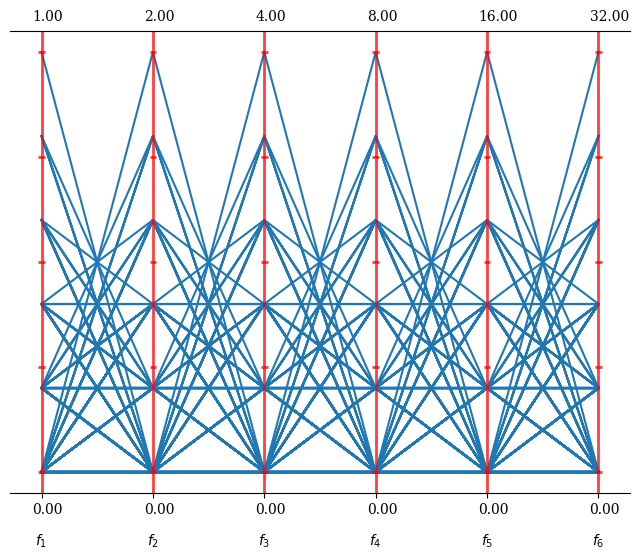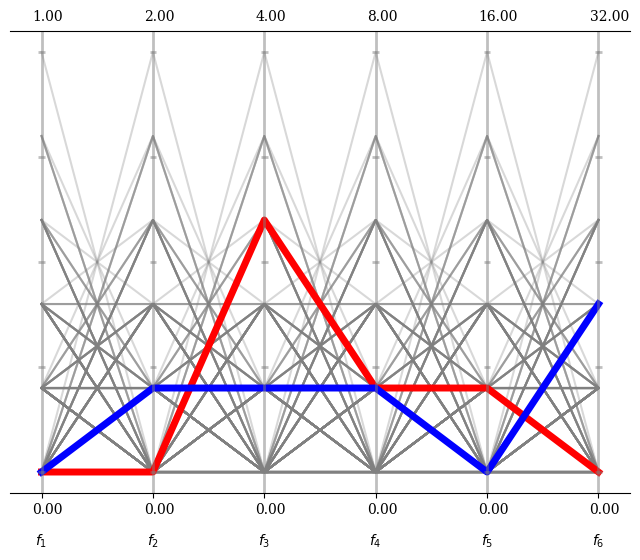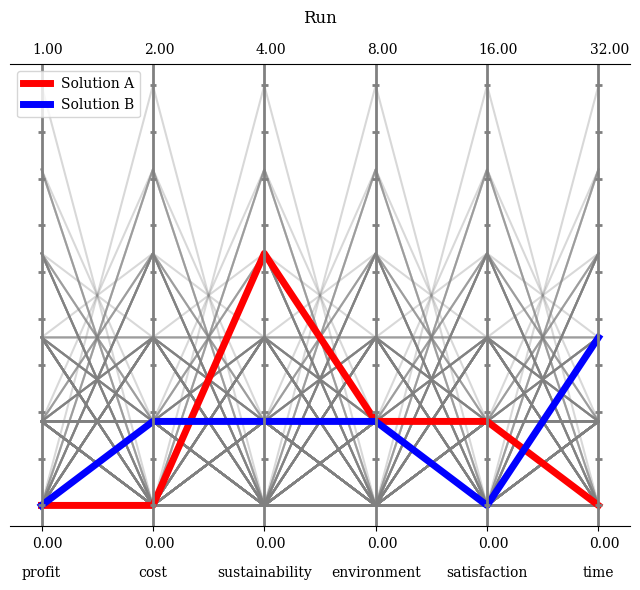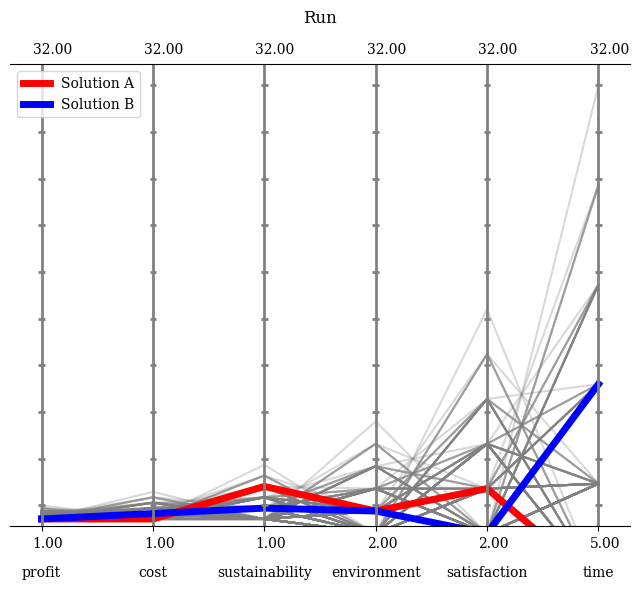Version: 0.5.0

Parallel Coordinate Plots¶

For higher-dimensional data, Parallel Coordinate Plots are a powerful technique to analyze how dense solutions are distributed in different ranges regarding each coordinate.

Let us create some data for visualization:

:
from pymoo.factory import get_problem, get_reference_directions
from pymoo.problems.many.dtlz import DTLZ1

ref_dirs = get_reference_directions("das-dennis", 6, n_partitions=5) * [2, 4, 8, 16, 32, 64]
F = DTLZ1().pareto_front(ref_dirs)

This is the Pareto-front for the DTLZ1 test problem with six objectives, with some scale added. We add a different scaling to show the effect of normalization later on. Let us assume our algorithm converged after some generations, and this is the result set.

:
from pymoo.visualization.pcp import PCP
:
<pymoo.visualization.pcp.PCP at 0x7f081fb46f10>This gives an idea of the overall result set. Let us assume we identified solution 50 and 75 to more of interest and like to highlight them in our plot:

:
plot = PCP()
plot.set_axis_style(color="grey", alpha=0.5)
plot.show()
:
<pymoo.visualization.pcp.PCP at 0x7f080df66e20>Please note that the PCP object just is a wrapper around a matplotlib figure. All options that apply for plotting the corresponding type (here plot, but it can also be scatter, polygon, …) can be used, such as linewidth, color or alpha.

Some more options to be used in a plot

:
n_ticks=10,
legend=(True, {'loc': "upper left"}),
labels=["profit", "cost", "sustainability", "environment", "satisfaction", "time"]
)

plot.set_axis_style(color="grey", alpha=1)
plot.show()
:
<pymoo.visualization.pcp.PCP at 0x7f080df66bb0>Moreover, if the boundaries should be set manually, this can be achieved by turning the default normalization of and providing them. Either directly as a NumPy array or just an integer to be set for all axes.

:
plot.reset()
plot.normalize_each_axis = False
plot.bounds=[[1,1,1,2,2,5],[32,32,32,32,32,32]]
plot.show()
:
<pymoo.visualization.pcp.PCP at 0x7f080df66bb0>
<Figure size 576x432 with 0 Axes>API¶

class pymoo.visualization.pcp.PCP(self, bounds=None, show_bounds=True, n_ticks=5, normalize_each_axis=True, bbox=False, **kwargs)

Parallel Coordinate Plot

Parameters
boundstuple

If plot requires normalization, it might be necessary to supply the boundaries. (Otherwise they might be approximate by the minimum and maximum of the provided data). The boundaries should be provided as a list/tuple or 2D numpy array, where the first element represents the minimum, second the second the maximum values. If only an integer or float is supplied, the boundaries apply for each variable.

axis_styledict

Most of the plots consists of an axis. The style of the axis, e.g. color, alpha, …, can be changed to further modify the plot appealing.

labelsstr or list

The labels to be used for each variable provided in the plot. If a string is used, then they will be enumerated. Otherwise, a list equal to the number of variables can be provided directly.

n_ticksint

Number of ticks to be shown on each parallel axis.

show_boundsbool

Whether the value of the boundaries are shown in the plot or not.

normalize_each_axisbool

Whether the values should be normalized either by bounds or implictly.

Other Parameters
figsizetuple

The figure size. Default (figsize=(8, 6)). For some plots changing the size might have side-effects for position.

titlestr or tuple

The title of the figure. If some additional kwargs should be provided this can be achieved by providing a tuple (“name”, {“key” : val}).

legendstr

Whether a legend should be shown or not.

tight_layoutbool

Whether tight layout should be used.

cmapcolormap

For some plots different kind of colors are used. The colormap can be changed to modify the color sequence for the plots.